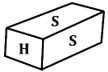Engineering Jobs   »   Civil Engineering Quiz

# RSMSSB-JE’21 CE: Daily Practices Quiz. 06-Sep-2021

As RSMSSB JE exam date 2021 is announced which is going to be held on 12th September 2021. As RSMSSB JE exam date 2021 is nearby so boost your preparation by attempt this Civil Engineering miscellaneous quiz for RSMSSB JE recruitment 2021.

Quiz: Civil Engineering
Exam: RSMSSB-JEn
Topic: Miscellaneous

Each question carries 1 mark
Negative marking: 1/3 mark
Time: 8 Minutes

Q1 A horizontal layer of bricks or stones is termed as a _________________.
(a) Course
(c) Stretcher
(d) Bed

Q2. What will be the shift of transition curve, if the length of transition curve is 80 m. and radius of the curve is 320 m?
(a) 0.011
(b) 0.83
(c) 0.89
(d) 21.33

Q3. In design of lacing system for a built-up steel column, the maximum allowable slenderness ratio of a lacing bar is
(a) 250
(b) 180
(c) 120
(d) 145

Q4. A survey done to understand the heavenly bodies is known as
(a) Celestial survey
(b) Astronomical survey
(c) Photographic survey
(d) Aerial survey

Q5. A cement concrete road is 1000 m long, 8 m wide and 15 cm thick over the sub-base of 10 cm thick gravel. The box cutting in road crust is ………
(a) 500 m³
(b) 1000 m³
(c) 1500 m³
(d) 2000 m³

Q6. Calculate the volume (cubic meter) of earth work in an embankment of length 15m. The top width of the embankment is 5 m and depth are 3m. The side slope is 1.5:1.
(a) 225
(b) 326.25
(c) 367
(d) 427.5

Solutions

S1. Ans.(a)
Sol. A horizontal layer of bricks or stones is termed as a course. The shorter face of the brick is called header and longer face of the brick is called stretcher.S = Stretcher

S2. Ans.(b)
Sol. Given,
Length of transition curve (L_s) = 80 m
Radius of the curve (R) = 320 m
Shift(s) = (L_s^2)/24R
=(80)^2/(24×320)
= 0.83 m

S3. Ans.(d)
Sol. In design of lacing system for a built-up steel column, the maximum allowable slenderness ratio of a lacing bar is 145.

S4. Ans.(b)
Sol. Astronomical survey → It is done to determine latitude, longitude, local mean time at any place on the earth surface. It is also done to understand heavenly bodies.

S5. Ans.(d)
Sol. The box cutting in road crust = 1000×8×(0.15+0.10) = 2000 m³.

S6. Ans.(d)
Sol. Given, Length = 15m.
Depth = 3m.
Width = 5m.
Side slope = 1.5:1 (H:V)
Volume of earth work = sectional Area × Length
= (BD+SD^2 )×L
=[5×3+1.5×(3)^2 ]×15
=427.5 m^3.

Sharing is caring!

Thank You, Your details have been submitted we will get back to you.